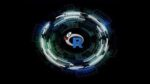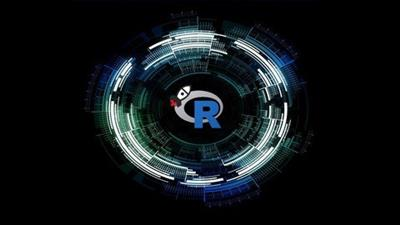Udemy – R for Data Science Your First Step as a Data Scientist

# Udemy – R for Data Science Your First Step as a Data Scientist

\$20.00

We only charge convenience fees

• Only Mega Links will be Provided
Guaranteed Safe CheckoutGenre: eLearning | MP4 | Video: h264, 1280×720 | Audio: AAC, 48.0 KHz
Language: English | Size: 5.38 GB | Duration: 10h 1m
Learn Data Science and Machine Learning (ML) with R Studio and submit your first Kaggle Project

What you’ll learn
Developing Linear Regression in R
Developing Logistic Regression in R
Learning how to Evaluate Data Science Models
Learning how to manipulate data with Dplyr
Building a Data Science Project end-to-end
Submit your own predictions into Kaggle
Description
So, you’ve learned a bit of R Basics and are looking to understand how R can be used for Data Science? And are looking for a course that explains all the theory behind algorithms with coding?
R is on of the de facto languages for a lot of Data Science projects today – either for enterprise-level projects or research, R is a modern and flexible language with a smooth learning curve that enables most professionals to build predictive models in quick fashion.
This course was designed to be your next step into the R programming world! We will delve deeper into the concepts of Linear and Logistic Regression, understand how Tree Based models work, learn how to evaluate predictive models and more. This course contains lectures around the following groups:
Code along lectures where you will see how we can implement the stuff we will learn!
Test your knowledge with questions and practical exercises with different levels of difficulty!
This course was designed to be focused on the practical side of coding in R – other than studying the functions that let us build algorithms automatically we will investigate deeply how models are trained and how they get to the optimum solution to solve our data science projects.
At the end of the course you should be able to use R in a data science context – understanding the choices you have to make when it comes to algorithms and learn how to evaluate those choises. Along the way you will also learn how to manipulate data with Dplyr because most of the times, in a Data Science project, more than half of time is spent on data preparation!
Here are some examples of things you will be able to do after finishing the course:
Solving Regression problems using Linear Regression or Regression Trees.
Solving Classification problems using Logistic Regression or Classification Trees.
Learn how to evaluate algorithms using different metrics.
Understanding the concept of bias and variance.
Using Random Forests and understanding the reasoning behind them.
Manipulating data using Dplyr.
Build your own Kaggle Data Science project!
Join thousands of professionals and students in this R journey and discover the amazing power of this statistical open-source language.

Homepage

``https://www.udemy.com/course/r-for-data-science-first-step-data-scientist/``